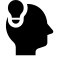# Longest Increasing Subsequence:From n's square to nlogn

## Algo demo with Python

Posted by viewsetting on November 2, 2018

# Definition

If a sequence is given as $a_1a_2…a_i….a_{n-1}a_n$ , then its Longest Increasing Subsequence is the longest seq whose elements are all in increasing order, every element should be greater (or equal) than its predecessor.

# $N^2$ Implement

Thinking about a DAG(Directed Acyclic Graph): if the element $a_i$ is considered now, which one would be the next in an increasing sequence started from $a_i$? Well, that would be $a_j$ for each $j$ larger than $i$ ,so is $aj$ , larger (or equal ) than $a_i$. If we give each $ai$ a node that store $len_i$ for the LIS ended by itself, then each $a_j$ that satisfied the restriction before, node of $a_j$ should be refreshed to a new value: $len_i +1$ . If we iterate that procedure, the LIS problem is solved.

def longest_increasing_subsequence(lst):
aid=[]
lst_in=[]
max_num=1
max_pos=0
for i in range (0,len(lst) ):
aid.append(1)
lst_in.append([lst[i]])

print("lst_in default:",lst_in)
for idx in range (0,len(lst)) :
for nxt in range (idx+1,len(lst)):
if lst[idx]<=lst[nxt] and aid[idx]+1>aid[nxt] :
aid[nxt]=aid[idx]+1
lst_in[nxt]=list(lst_in[idx])   #without list() stuff gone wild!
lst_in[nxt].append(lst[nxt])
if aid[nxt]>max_num :
max_num=aid[nxt]
max_pos=nxt

print('array of aid : ',lst_in)
return max_num,lst_in[max_pos]

lst=[5,1,45,2,5,7,8,1]
print('lst :',lst)
print('len_of_LIS & LIS :',longest_increasing_subsequence(lst))# $N\log N$ Implement

Considering a case that two LIS that share the same length, which should be more potential to be the LIS of the whole seq? U can conclude that it must be the one which ended with the smaller number. Because in this situation,it does not matter if a number with smaller value appears later than a previous larger one. Only value of the node is the key to construct an increasing sequence. Therefore we can use another list or array $dp_i$, which means the end number of the LIS whose size is $i$ . We can simply scan the original seq $a$,then if $a_i$ is larger than the latest largest $dp_k$ ,update $dp_{k+1}$ as $a_i$ . If not, use binary search to find a $dp_J$ that is the smallest one larger than $a_i$, replace it with $a_i$.

import bisect
def bin_LIS(lst):
dp=[0XFFFFFFFFF]*(len(lst)+1)
node=[[]*len(lst)]*len(lst)
leng=0
dp=lst
node=[lst]
print ('iteration: ',0,': ',node)
for i in range (1,len(lst)):
if(dp[leng]<lst[i]):
dp[leng+1]=lst[i]
leng=1+leng
node[leng]=list(node[leng-1])
node[leng].append(lst[i])
else:
pt=bisect.bisect_left(dp,lst[i])
dp[pt]=lst[i]
node[pt][len(node[pt])-1]=lst[i]
print ('iteration: ',i,': ',node)
return dp,leng+1,node

lst=[5,1,4,2,5,0,7,2,10,7,8,1,11]
bin_LIS(lst)